# What is a Mixed Number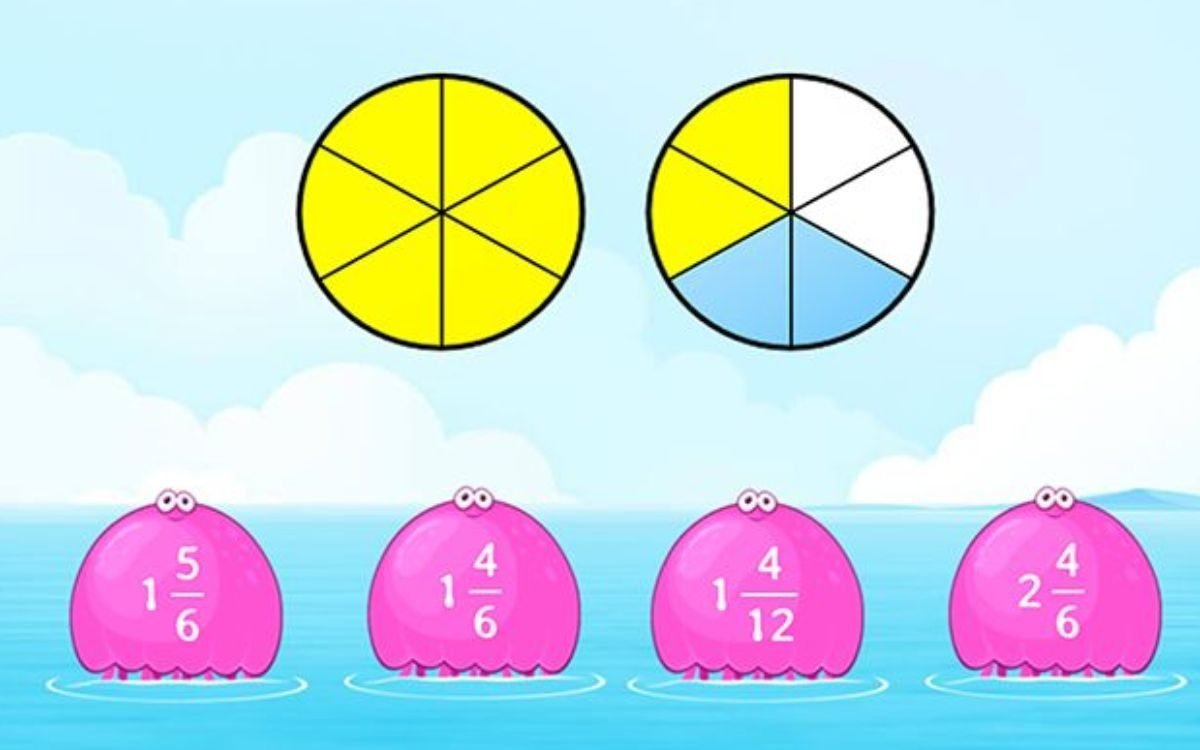While doing the question and solving the problems we all are facing some problems with the terms. There are different names for the different terms in maths. But what is a mixed number? This we have seen so many times but specifically with the name we never heard actually. Here are some examples of mixed numbers. And how they are different from the others.

## How to Write a Mixed Number?

A mixed number is the combination of the whole no and the fraction. They generally align between the two whole no on the number line. Basically, there are two types of fractions.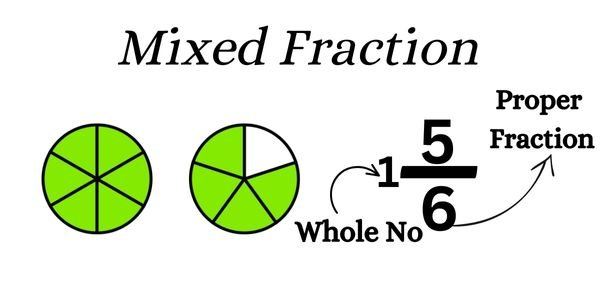The first one is the Proper fraction and the second one is the improper fraction.

## Proper Fraction

Fraction is said to be a proper fraction if the numerator is smaller than the denominator. This is when one thing is divided properly according to the parts it has.

For example, we have divided one thing into six parts and taken out the two from them. The fraction will be 2/6.

Here the numerator (the no written above the fraction) is smaller than the denominator.

## Improper Fraction

Fraction is the improper fraction if the numerator is larger than the denominator. We have taken out more parts from the one thing which we have divided.

For example, We have divided one thing into the Eleven-part but taken out the eight-part from it. Then the fraction will be 11/6.

Here the numerator is larger than the denominator.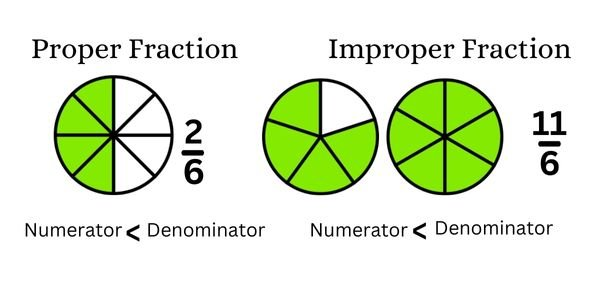The Improper fraction can only be converted to the Mixed fraction. This we do to make the improper faction the proper fraction.

## How to Convert Improper Fraction Into Mixed Fraction

To convert the improper fraction into the proper fraction. We take the help of the mixed fraction form. When the fraction is in the improper form and is considered to be incomplete because the right form is the proper fraction.

If you want to convert the improper fraction into the mixed fraction. You just need to do the division. The denominator is the divider. And the numerator is the dividend. So, divide the numerator by the denominator. The proper dividend you get is the whole no and the reminder is now the new denominator.

Let’s see the step by step process how we can convert it.

Example 1: Convert the Fraction 11/6 into the mixed fraction.Sol: Take the Fraction given and divide it. Here you will get these ans

• Dividend:1
• Reminder:5

Now arrange the numbers. The dividend you are getting will be at the place of the whole no. The reminder will be at the numerator place and the previous denominator will be your same denominator. Look at the picture for more clarification.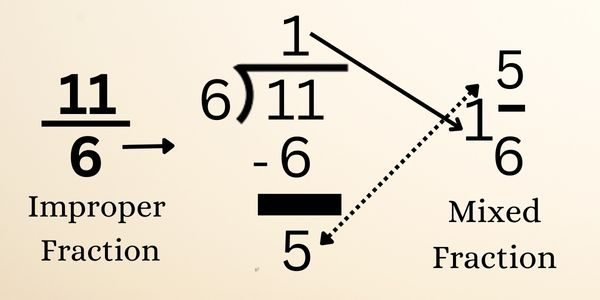## How to Convert Mixed Fraction into Improper Fraction

We convert the mixed fraction into the improper fraction because we cannot add, subtract, multiply, or divide the mixed fraction directly. To convert the mixed fraction into the improper fraction we need to follow some steps and remember the step carefully.

Steps to convert the mixed fraction into the improper fraction

• Multiply the whole no and the denominator
• Then add the numerator to the and of multiplication result.
• The and will be at the numerator place
• Write the denominator the same as it was actually

Let’s take an example to understand it more clearly.

Example: Convert the mixed Fraction 1 5/6 into the improper fraction.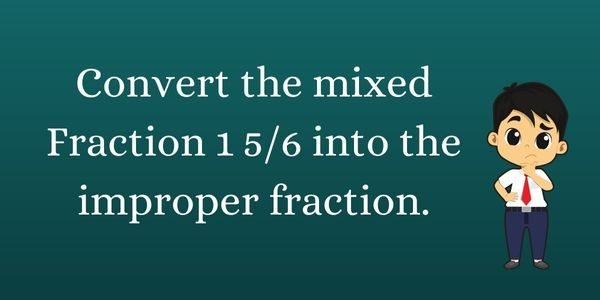Sol: Write the Mixed fraction and multiply its denominator with the whole number. And Add the numerator value to the ans. The ans will be your new numerator and the denominator will be the same value as the previous one. For more clarity, you can see the image.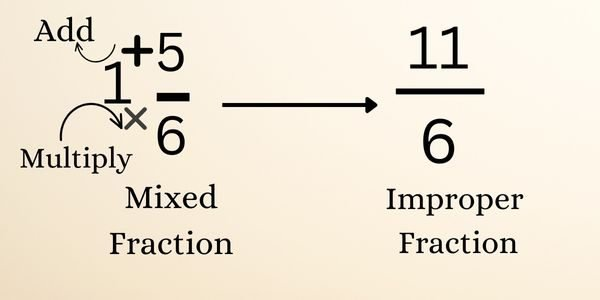For addition, subtraction, multiplication, and division we need to convert the mixed fraction into the improper fraction. Once it gets converted into that form we can simpally you can do the calculation. But after that, you need to convert the improper fraction into the mixed fraction again.

Also Read: How to Convert SGPA into Percentage

What is the Average Weight of a 12-Year-Old

Why do Dogs Have Whiskers?

What breed is my cat

What Companies are in The Consumer Services Field?